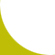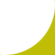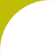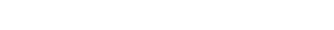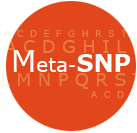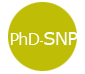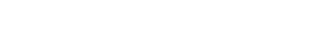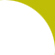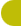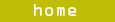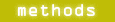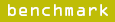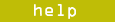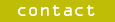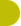Meta-SNP
Meta-predictor of disease causing variants

Introduction
In recent years the number of human genetic variants deposited into the publicly available databases has been increasing exponentially. The latest version of dbSNP, for example, contains ~50 million validated Single Nucleotide Variants (SNVs). SNVs make up most of human variation and are often the primary causes of disease. The non-synonymous SNVs (nsSNVs) result in single amino acid substitutions and may affect protein function, often causing disease. Although several methods for the detection of nsSNV effects have already been developed, the consistent increase in annotated data is offering the opportunity to improve prediction accuracy.
Here we present a new approach for the detection of disease-associated nsSNVs (Meta-SNP) that integrates four existing methods: PANTHER, PhD-SNP, SIFT and SNAP . Meta-SNP predictor is made avalible through a docker container at the link https://hub.docker.com/r/biofold/meta-snp.

Methods
We trained Meta-SNP, a random forest-based binary classifier to discriminate between disease-related and polymorphic non-synonymous SNVs. Meta-SNP takes as input the output of the four predictors described above as an eight-element feature vector composed of two groups of four elements each (see figure). The first group is the set of raw output scores of the variant predictions from PANTHER, PhD-SNP, SIFT and SNAP. In case one of the input methods does not return a prediction, we used the method-defined default threshold for differentiating neutrals and non-neutrals as input to Meta-SNP (SNAP=0, SIFT=0.05, PhD-SNP=0.5, PANTHER=0.5).
The second group contains four elements extracted from the PhD-SNP protein sequence profile: (1 and 2) frequencies of the wild-type (Fwt) and mutant (Fmut) residues in the mutated site, (3) the total number of sequences aligned at the mutated site (Nal) and (4) the conservation index (CI) . Sequence profile information modulates Meta-SNP predictions by the conservation of the mutated position. This information is redundant across the four component methods, so for Meta-SNP we used only one version of the sequence profile - that from PhD-SNP.
Meta-SNP is a 100-tree RandomForest WEKA  library implementation, trained on SV-2009 using 20-fold cross-validation. The predictor outputs the probability that a given nsSNV is disease-related, where scores >0.5 indicate that the given the variant is disease-causing.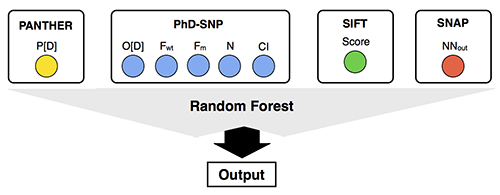Results
To improve the detection of deleterious variants, we developed a meta-predictor (Meta-SNP) that combines the outputs of PANTHER, PhD-SNP, SIFT and SNAP. Meta-SNP uses single predictor outputs as in input; it was trained and tested on the SV-2009 dataset using a 20-fold cross-validation procedure. Meta-SNP reaches 79% overall accuracy, 0.59 MCC and 0.87 AUC resulting in better performance than each single method (Table 1).

 Methods Q2 P(D) Q(D) P(N) Q(N) MCC AUC PANTHER 0.74 0.79 0.73 0.69 0.74 0.82 0.74 PhD-SNP 0.76 0.78 0.74 0.75 0.78 0.53 0.84 SIFT 0.70 0.74 0.64 0.68 0.76 0.41 0.73 SNAP 0.64 0.59 0.90 0.79 0.38 0.33 0.79 Meta-SNP 0.79 0.80 0.79 0.79 0.80 0.59 0.87

The ability of the meta-predictor approach to select high reliable prediction has been proved calculating the accuracy of Meta-SNP on the subsets composed by cases where all the predictions are in agreement (Consensus), one of the two possible classes is in majority (Majority) and when half of the methods predict one Disease and the other half Neutral (Tie). The results shows that the accuracy of Meta-SNP increases from the Tie to the Consensus subset (Table 2).

 Datasets Q2 P(D) Q(D) P(N) Q(N) MCC AUC DB All 0.79 0.80 0.79 0.79 0.80 0.59 0.87 100 Consensus 0.87 0.88 0.92 0.87 0.80 0.73 0.91 46 Majority 0.75 0.72 0.64 0.76 0.82 0.47 0.82 40 Tie 0.69 0.62 0.57 0.73 0.76 0.34 0.75 14

The overall accuracy Q2 is:

Q2=p/N

where p is the total number of correctly predicted residues and N is the total number of residues.
The correlation coefficient MCC is defined as:

C(s)=[p(s)n(s)-u(s)o(s)] / W

where W is the normalization factor

W=[(p(s)+u(s))(p(s)+o(s))(n(s)+u(s))(n(s)+o(s))]1/2

for each class s (D and N, for disease-related and polymorphism, respectively); p(s) and n(s) are the total number of correct predictions and correctly rejected assignments, respectively, and u(s) and o(s) are the numbers of under and over predictions.

The coverage for each discriminated structure s is evaluated as:

Q(s)=p(s)/[p(s)+u(s)]

where p(s) and u(s) are as defined above. The probability of correct predictions P(s) (or accuracy for s) is computed as:

P(s)=p(s)/[p(s) + o(s)]

where p(s) and o(s) are defined above (ranging from 1 to 0).

References

 Capriotti E, Altman RB, Bromberg Y (2013). Collective judgment predicts disease-associated single nucleotide variants. mutations in proteins. BMC Genomics. Suppl 3: S2.
 Pei J, Grishin NV. (2001) AL2CO: calculation of positional conservation in a protein sequence alignment. Bioinformatics. 17(8):700-712.
 Hall M, Frank E, Holmes G, Pfahringer B, Reutemann P, Witten IH. (2009) The WEKA Data Mining Software: An Update. ACM SIGKDD Explorations. 11:10-18.